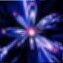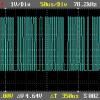# Rk4 1 Equation

6 replies to this topic

### #1girdeux

girdeux

•• Members
•• 88 posts
• Location:Spain / Castell?n

• Calculators:
casio fx-115ms;

Posted 11 May 2008 - 06:55 PM

I want to solve only one equation by RK4Rich function, but doesn't work ok.

```require("LNA/RK4Rich")

T0=293
t0=0
tf=40*60

local function RHS(t,T)
return {5.08*10^-12*((T0+0.41*t)^4-T^4)+(2.75*10^4+52.16*10^-3/(T0+0.41*t)^0.5)*(T0+0.41*t-T)}
end

local tp,Tp
tp,Tp=RK4Rich(RHS,t0,{T0},tf,true,5*10^-4)

print(#tp.." puntos de integracion.")```

the function it's rigth, you can comprobe it with mathematica:

```T0 = 293;
t0 = 0;
tf = 40*60;
solution=NDSolve[{T'[t]==
5.08*10^-12*((T0+0.41*t)^4-T[t]^4)+(2.75*10^4+52.16*10^-3/(T0+0.41*t)^0.5)*(T0+0.41*t-T[t])}
,T,{t,t0,tf}];
Plot[T[t] /. solution, {t, t0, tf}];```

why??

### #2girdeux

girdeux

•• Members
•• 88 posts
• Location:Spain / Castell?n

• Calculators:
casio fx-115ms;

Posted 22 May 2008 - 09:18 PM

### #3ECE

ECE

Casio Freak

•• Members
•• 152 posts
• Gender:Not Telling
• Location:USA

• Calculators:
fx-CG20 o.s1.04
fx9860GII SD o.s2.00
fx9860G Slim o.s1.10
fx9860GSD o.s2.01 (GII hacked)
Algebra FX2.0 Plus
CFX9850GBplus
fx5800p
fx3650p
fx570ES Plus
EA-200 Casio Data Analyzer

Posted 23 May 2008 - 02:07 PM

Hi,
?I want to solve only one equation by RK4Rich function? did you mean runge-kutta order 4 for solving ordinary differential equation method numerically, then what is the ?Rich function?? please explain without the abbreviations so maybe some one can help you.

### #4PAP

PAP

Casio Overlord

•• Members
•• 681 posts
• Gender:Male
• Location:Somewhere in Europe.
• Interests:Computer Algebra, Numerical Analysis.

• Calculators:
ClassPad 300 (plus an old Casio model, with only a few Kb ram).

Posted 23 May 2008 - 09:00 PM

I want to solve only one equation by RK4Rich function, but doesn't work ok.

Sorry for answering so late, I'm actually too busy at the moment...

I think RK4Rich does not work properly because you forgot the output argument y, which is mandatory; you just used the optional output, but mandatory output should be present as well, even if you don't need it. Try this:
`T,tp,Tp=RK4Rich(RHS,t0,{T0},tf,true,5*10^-4)`
I bet RK4Rich will work then. If not, double-check your RHS function, and make sure it implements the right-hand side of the differential equation you want to solve.

then what is the ?Rich function?? please explain without the abbreviations so maybe some one can help you.

"Rich" is not a function written by girdeuax, it's part of the name of the LNA function. "Rich" comes from "Richardson extrapolation". Remember, RK4Rich is not just an implementation of the Runge-Kutta method of fourth order; it also uses adaptive stepsize control (via Richardson extrapolation). Runge-Kutta method without stepsize control is not available in LNA, and it will never be, since constant integration step is a common source of inaccurate results.

Anyway, your question should be posted at the LNA topic, not here....

### #5ECE

ECE

Casio Freak

•• Members
•• 152 posts
• Gender:Not Telling
• Location:USA

• Calculators:
fx-CG20 o.s1.04
fx9860GII SD o.s2.00
fx9860G Slim o.s1.10
fx9860GSD o.s2.01 (GII hacked)
Algebra FX2.0 Plus
CFX9850GBplus
fx5800p
fx3650p
fx570ES Plus
EA-200 Casio Data Analyzer

Posted 24 May 2008 - 10:23 AM

thanks.

### #6girdeux

girdeux

•• Members
•• 88 posts
• Location:Spain / Castell?n

• Calculators:
casio fx-115ms;

Posted 25 May 2008 - 11:12 AM

Yes the function was wrong written jeje (a - sign was missed)

### #7PAP

PAP

Casio Overlord

•• Members
•• 681 posts
• Gender:Male
• Location:Somewhere in Europe.
• Interests:Computer Algebra, Numerical Analysis.

• Calculators:
ClassPad 300 (plus an old Casio model, with only a few Kb ram).

Posted 26 May 2008 - 09:43 PM

Yes the function was wrong written jeje (a - sign was missed)

You need to try harder to find a bug in LNA, my friend(but feel free to ask if in doubt, just remember to post in the right topic)

#### 0 user(s) are reading this topic

0 members, 0 guests, 0 anonymous users